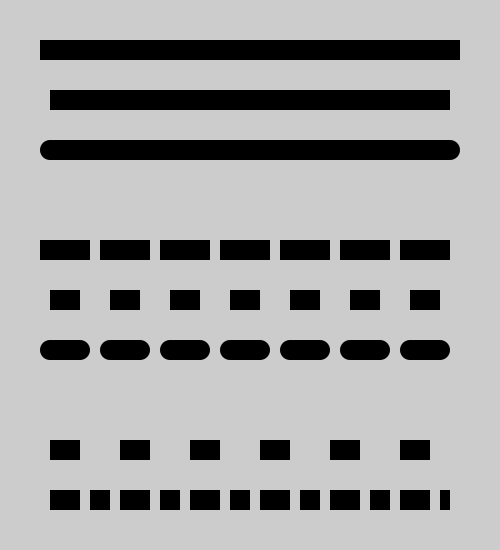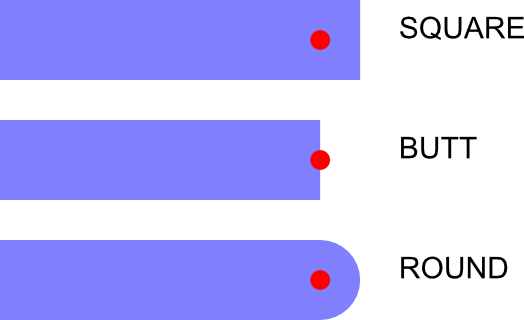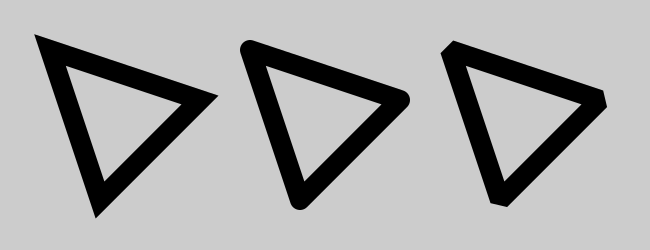# Stroke styles in generativepy

Martin McBride, 2021-12-12
Tags generativepy tutorial fill stroke rectangle
Categories generativepy generativepy tutorialThis tutorial describes how to style the lines used to stroke a shape. This includes:

• The style of the line caps.
• Applying a dash pattern.
• The style of the line joins.

You should read the main fill and stroke article before this one.

## Line styles

Here is a sample Python program for creating lines with various line end and dash styles:

from generativepy.drawing import make_image, setup, SQUARE, BUTT, ROUND, BEVEL, MITER
from generativepy.color import Color
from generativepy.geometry import Rectangle, Line, Triangle

def draw2(ctx, pixel_width, pixel_height, frame_no, frame_count):
setup(ctx, pixel_width, pixel_height, background=Color(0.8))

black = Color(0)

Line(ctx).of_start_end((50, 50), (450, 50)).stroke(black, 20, cap=SQUARE)
Line(ctx).of_start_end((50, 100), (450, 100)).stroke(black, 20, cap=BUTT)
Line(ctx).of_start_end((50, 150), (450, 150)).stroke(black, 20, cap=ROUND)

Line(ctx).of_start_end((50, 250), (450, 250)).stroke(black, 20, cap=SQUARE, dash=)
Line(ctx).of_start_end((50, 300), (450, 300)).stroke(black, 20, cap=BUTT, dash=)
Line(ctx).of_start_end((50, 350), (450, 350)).stroke(black, 20, cap=ROUND, dash=)

Line(ctx).of_start_end((50, 450), (450, 450)).stroke(black, 20, cap=BUTT, dash=[30, 40])
Line(ctx).of_start_end((50, 500), (450, 500)).stroke(black, 20, cap=BUTT, dash=[30, 10, 20, 10])

make_image("stroke-style-tutorial.png", draw2, 500, 550)


This code is available on github in tutorial/shapes/fill-stroke.py.

Here is the resulting image:## Line cap styles

This section of the code draws three lines with different end styles:

    Line(ctx).of_start_end((50, 50), (450, 50)).stroke(black, 20, cap=SQUARE)
Line(ctx).of_start_end((50, 100), (450, 100)).stroke(black, 20, cap=BUTT)
Line(ctx).of_start_end((50, 150), (450, 150)).stroke(black, 20, cap=ROUND)


This code draws the top three lines in the original diagram.

Each line is 400 units long and 20 units wide. However, the line cap increases the length of some lines slightly. This diagram shows each case:For each line, the red dot indicates the nominal line endpoint.

For a line with SQUARE caps, the line is extended beyond the endpoint by half the line width. This happens at both ends of the line.

For a line with BUTT caps, the line ends exactly at the endpoint. This is the default. The SQUARE case and the BUTT case look very similar, except that the SQUARE case creates a slightly longer line.

For a line with ROUND caps, the line is extended beyond the endpoint using a semicircle with a radius of half the line length. Again, this happens at both ends of the line.

## Line dash styles

This section of the code draws five dashed lines:

    Line(ctx).of_start_end((50, 250), (450, 250)).stroke(black, 20, cap=SQUARE, dash=)
Line(ctx).of_start_end((50, 300), (450, 300)).stroke(black, 20, cap=BUTT, dash=)
Line(ctx).of_start_end((50, 350), (450, 350)).stroke(black, 20, cap=ROUND, dash=)

Line(ctx).of_start_end((50, 450), (450, 450)).stroke(black, 20, cap=BUTT, dash=[30, 40])
Line(ctx).of_start_end((50, 500), (450, 500)).stroke(black, 20, cap=BUTT, dash=[30, 10, 20, 10])


This code draws the bottom five lines in the original diagram.

The dash parameter is a list of numbers specifying the lengths of the dashes and spaces.

In the first three lines, dash is set to . This means that each dash and each space is 30 units.

You would expect this to create a line where the dashes and spaces are equal. However, each line section has line caps added. For line caps of SQUARE or ROUND, this means that the dash is extended into the space, making the dashes appear longer than the spaces. The second line has BUTT caps, so the dashes and spaces are indeed the same length.

In the fourth line, the dash pattern is [30, 40], meaning the dashes are 30 units long and the spaces 40.

In the fifth line, the dash pattern is [30, 10, 20, 10]. This gives a pattern of dash 30, space 10, dash 20, space 10, that repeats along the line.

## Line join styles

In addition to line caps, we can also control the style of line joins. Here is some code to illustrate this:

def draw3(ctx, pixel_width, pixel_height, frame_no, frame_count):
setup(ctx, pixel_width, pixel_height, background=Color(0.8))

black = Color(0)

Triangle(ctx).of_corners((50, 50), (200, 100), (100, 200)).stroke(black, 20, join=MITER)
Triangle(ctx).of_corners((250, 50), (400, 100), (300, 200)).stroke(black, 20, join=ROUND)
Triangle(ctx).of_corners((450, 50), (600, 100), (500, 200)).stroke(black, 20, join=BEVEL)

make_image("join-style-tutorial.png", draw3, 650, 250)


This code draws three triangles, with different join styles, controlled by the join parameter of the stroke method. Here is the result:MITER gives a pointed corner, ROUND gives a rounded off corner, BEVEL is similar to MITER but with the point removed.

## Miter limit

A feature of the MITER style is that the point gets longer as the angle between the two lines gets smaller. If two lines join at a very small angle, the miter length can be very long and that can look odd.

To avoid this, whenever two lines have an angle of less than approximately 11 degrees, the join will be drawn as a BEVEL rather than a MITER, so the long point is removed.

We can control this using the miter_limit parameter of stroke. Some example values are:

Value Cutoff angle
0 Always use bevel
1.414 Use bevel if angle less than 90 degrees
2.0 Use bevel if angle less than 60 degrees
10.0 (default) Use bevel if angle less than about 11 degrees
1000.0 Use bevel if angle less than about 0.1 degrees

There is no option for turning the mitre limit off, but setting a large value will effectively disable it for most angles.

## Pattern fills

A stroke is painted as a shape in its own right. This means that you can fill it with a pattern (such as a gradient) rather than a solid colour. See patterns for more information.

If you found this article useful, you might be interested in the book Computer Graphics in Python or other books by the same author.

#### Popular tags

2d arrays abstract data type alignment and angle animation arange arc array arrays behavioural pattern bezier curve built-in function callable object chain circle classes clipping close closure cmyk colour combinations comparison operator comprehension context context manager conversion count creational pattern data science data types design pattern device space dictionary drawing duck typing efficiency ellipse else encryption enumerate fill filter font font style for loop function function composition function plot functools game development generativepy tutorial generator geometry gif global variable gradient greyscale higher order function hsl html image image processing imagesurface immutable object in operator index inner function input installing iter iterable iterator itertools join l system lambda function len line linear gradient linspace list list comprehension logical operator lru_cache magic method mandelbrot mandelbrot set map matplotlib monad mutability named parameter numeric python numpy object open operator optimisation optional parameter or pandas partial application path pattern permutations polygon positional parameter print pure function python standard library radial gradient range recipes rectangle recursion reduce repeat rgb rotation roundrect scaling scipy sector segment sequence setup shape singleton slice slicing sound spirograph sprite square str stream string stroke structural pattern subpath symmetric encryption template text text metrics tinkerbell fractal transform translation transparency triangle truthy value tuple turtle unpacking user space vectorisation webserver website while loop zip